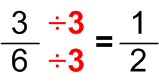# Simplifying numerical fractions

Simplifying numerical fractions is a very usefull skill and knowing how to make fractions simpler can make a world of diference. If you are solving an equation in which there are fractions with large numerators or denominators, reducing those fractions to their lowest value will make finding the result much easier.  In order to make a fraction simpler, the fraction needs to have at least two numbers that could be divided with same number. For example; you have a fraction 20/40 that can be simplified because both numerator and denominator could be divided with same number (in this case, it is number 20). In the end, you will get the number 1/2 as the result. Dividing both the numerator and the denominator with the same number is very important since it is the only way their ratio will not change and therefore influence the end result. If you want to simplify the fraction as much as possible, the number you use for simplifycation should be the greatest common factor of the numerator and the denominator.

For practice, you can use the worksheets below. They should provide you with enough practice material to help you master this skill as soon as possible.## Simplifying numerical fractions exams for teachers

 Exam Name File Size Downloads Upload date Proper fractions Simplifying proper fractions – easy 0 B 2446 January 1, 1970 Simplifying proper fractions – medium 0 B 1847 January 1, 1970 Simplifying proper fractions – hard 0 B 1984 January 1, 1970 Mixed numbers Simplifying mixed numbers – easy 0 B 2321 January 1, 1970 Simplifying mixed numbers – medium 0 B 1544 January 1, 1970 Simplifying mixed numbers – hard 0 B 1797 January 1, 1970 Simplifying improper fractions – easy 0 B 1790 January 1, 1970 Improper fractions Simplifying improper fractions – medium 0 B 1860 January 1, 1970 Simplifying improper fractions – hard 0 B 1569 January 1, 1970 Simplifying improper fractions into mixed numbers – easy 0 B 1403 January 1, 1970 Simplifying improper fractions into mixed numbers – medium 0 B 1368 January 1, 1970 Simplifying improper fractions into mixed numbers – hard 0 B 1618 January 1, 1970

## Simplifying numerical fractions worksheets for students

 Worksheet Name File Size Downloads Upload date Simplifying proper fractions 0 B 1936 January 1, 1970 Simplifying mixed numbers 0 B 1757 January 1, 1970 Simplifying improper fractions into mixed numbers 0 B 1354 January 1, 1970 Simplifying improper fractions 0 B 1503 January 1, 1970